Mobile QR Code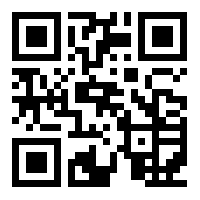1. (College of art and design, Luoyang Institute of Science and Technology, Luoyang, 471003, China)

K-means algorithm, Deep learning, Oracle images, Self-defined classification, Transfer learning, Convolutional neural network

## 1. Introduction

Oracle bone inscriptions are the earliest known mature writing system in China and East Asia. The inscriptions are engraved on tortoise shells and animal bones . There are some drawbacks in the traditional method for ancient text interpretation, and the efficiency of text interpretation is not high . First of all, researchers need to have much professional knowledge of oracle bone inscriptions . Second, the traditional methods only report'' from the isolated rubbing information<note: ambiguous>, they do not know the semantics of the oracle bone inscriptions, and they lack context information .

<New paragraph>With the digitization of ancient characters, computer technology provides a powerful tool for oracle research . With the enrichment of a large number of oracle digital research materials, the scale of basic oracle data is increasing day by day, and the systematic oracle data provides a guarantee<note: ambiguous>for the computer methods for oracle research. In addition to the oracle text itself, it is also very important to analyze the oracle data . Therefore, in order to improve the efficiency of oracle research, many researchers have effectively classified images and achieved some results.

One study  proposed a method of oraclebone character recognition based on cross modal depth measurement learning. In order to improve the performance of rubbing oracle bone character recognition, the method was based on cross modal depth measurement learning. Shared feature space modeling and nearest neighbor classification was done on copies of an oracle bone character and rubbing oracle bone character<note: ambiguous>for cross-modal recognition. This method is significantly better than the single-mode method in the task of rubbing oracle bone character recognition. At the same time, it can also recognize a new type of rubbing oracle bone characters incrementally. There is much research on this method of recognition of oracle images, and the classification is the key, but more classification research is needed.

Another study  proposed a method of oracle component recognition based on capsule network oracle bone inscriptions. As the earliest formed writing system in China, the inscriptions have important cultural and academic value. The study of oracle components and their configuration system is important for deciphering unrecognized oracle inscriptions, but the marking of oracle components can only be completed by senior experts, and it takes much time and energy to solve these problems. A model called Oraclenetwas proposed based on a capsule network and transfer learning, which can automatically identify and mark the components contained in oracle texts. Oracle glyphs and component datasets containing markers were constructed for model training and evaluation. The prediction accuracy of this method for oracle components is more than 60%, and the accuracy of top5 is 71.56%<note: ambiguous>, which verifies the effectiveness of the model. However, the operation process of this method is cumbersome and feasible.

<New paragraph>Another study  provides a deep learning-based method for ancient Japanese character recognition. This method binarizes an image containing ancient text, fills the text with black, fills the non-text part with white, and uses the density and layout of the black part for judgment and classification. Experiments were carried out with AlexNet's test dataset, and the results showed that this method can more accurately identify ancient Japanese characters.

After studying the research on other classification methods, we designed an automatic classification method fororacle images based on deep learning. Through a deep learning artificial intelligence algorithm, we improved the effectiveness and efficiency of oracle image classification. The main technical route is as follows:

Step 1: Determine the clustering center of an oracle image using the k-means algorithm, take the obtained oracle image block as parallel input, extract a dictionary, output the oracle image features through a feature mapping function, and realize the feature extraction of an oracle image.

Step 2: The dimension of the oracle image is reduced by the compressed sensing (CS) algorithm, and the oracle feature’s compressed sensing signal is sparse under a certain base to realize the preprocessing of image features.

Step 3: Use the centers of six feature points in a feature to describe the center of the feature points of the whole oracle image, determine the position change state of the feature points, and characterize the key features of the imageto realize the target alignment of the feature points of the oracle image. Based on this, the image feature data are learned using a transfer learning model. An automatic classifier of oracle images is constructed through a convolution neural network to realize the automatic classification of oracle images.

Step 4: Experimental analysis.

Step 5: Conclusion.

In this paper, not only are k-means and CS algorithms used, the average value of feature point coordinates is used to avoid regional overlap. The threshold center point is used to control the position change of feature points, thus reducing the classification error of target points.

## 2. Feature Extraction and Preprocessing of Oracle Bone Inscriptions

### 2.1 Research on Feature Extraction Method of Oracle Image

Feature extraction of oracle images provides an effective basis for the classification of oracle images . We achieved feature extraction using the k-means algorithm. In the field of computer vision, the k-means algorithm is mainly used to construct a word bag of visual vocabulary, which has been widely used because of its simplicity and efficiency . The k-means algorithm is a clustering method based on similarity measurement between samples and type of unsupervised learning method. When doing classification assignments, the k-means algorithm initializes a group of cluster centers and determines what sample point belongs to which cluster center by calculating the distance from other sample points to the cluster center. The algorithm collects the features of the research object to realize feature extraction. Its basic principle is shown in Fig. 1.

The k-means clustering algorithm takes the sum of squares of errors as a criterion function and modifies the clustering center point by point. It assigns the sample to a group according to a certain calculation method for each sample and then recalculates the mean value of the group. At the same time, the new mean value is used as the new clustering center of the group for the next sample clustering. In the feature extraction and summary of oracle images, the clustering centers of koracle images are initialized randomly, and the clustering center set of clustered oracle image features is obtained as follows:

##### (1)
$A=\left\{a_{1},a_{2},\ldots a_{n}\right\}$

In Eq. (1), $A$ represents the clustering center set, and $a_{1},a_{2},\ldots a_{n}$ represent cluster centers. For sample i in the cluster center set of oracle-image features, it calculates the category that the feature point should be assigned to:

##### (2)
$\mathrm{b}^{\left(\mathrm{i}\right)}=\text{argmin}\left\| \mathrm{b}_{\mathrm{i}}-\mathrm{a}_{\mathrm{i}}\right\| ^{2}$

In Eq. (2), $\left\| \mathrm{b}_{\mathrm{i}}-\mathrm{a}_{\mathrm{i}}\right\| ^{2}$ is the distance between the target sample and cluster centers. $\mathrm{b}^{\left(\mathrm{i}\right)}$ is its minimum value. Again, for each class j of the oracle image feature cluster center, the cluster center is recalculated again:

##### (3)
$c_{j}=\frac{\sum _{i=1}^{m}\left\{b^{\left(i\right)}=j\right\}}{\sum _{j=1}^{n}\left\{b^{\left(i\right)}=j\right\}d^{\left(i\right)}}$

Finally, according to the determined feature clustering center of the oracle image, the features of the oracle image are extracted. Image blocks are randomly extracted from the input oracle image. This operation is irrelevant to the images, so it can be carried out in parallel. At the same time, in order to reduce redundant information and retain key information, the extracted image blocks are preprocessed, including normalization and whitening processes. The preprocessing can also be carried out in parallel among multiple map nodes to improve efficiency. Finally, the obtained image block is used as the input of the parallelized k-means algorithm to extract the dictionary. After obtaining the dictionary, the image is mapped into a new expression of image features through the selected feature mapping function. The feature extraction process of an oracle image is shown in Fig. 2.

In the extraction process of Fig. 2, after learning the dictionary D in the feature extraction, the feature mapping function is defined, which can map an input vector into a new feature expression in dimension K, in which $\mathrm{v}\left(\mathrm{z}\right)$ is the mean of element z. The feature mapping function outputs the extracted oracle image features as Eq. (4):

##### (4)
$\mathrm{p}\left(\mathrm{x}\right)=\max \left\{0,\mathrm{v}\left(\mathrm{z}\right)-\mathrm{v}_{\mathrm{k}}\right\} \\$
##### (5)
$\mathrm{v}_{\mathrm{k}}=\left\| \mathrm{x}-\mathrm{d}^{\mathrm{k}}\right\| _{2}$

$\mathrm{p}\left(\mathrm{x}\right)$ represents the output oracle image feature result, $\mathrm{v}_{\mathrm{k}}$ represents the cluster center distance, and $\mathrm{d}^{\mathrm{k}}$ represents the sparse coding value.

In the feature extraction of an oracle image, the clustering center of the oracle image is determined with the help of the k-means algorithm. The obtained oracle image block is used as parallel input to extract the dictionary. The oracle image features are output through the feature mapping function to realize the feature extraction of the oracle image.

##### Fig. 1. Diagram of oracle image features with k-means.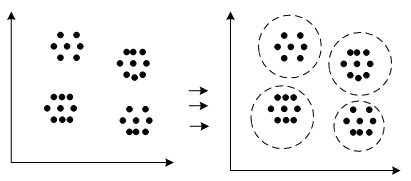##### Fig. 2. Oracle bone text image-feature extraction process.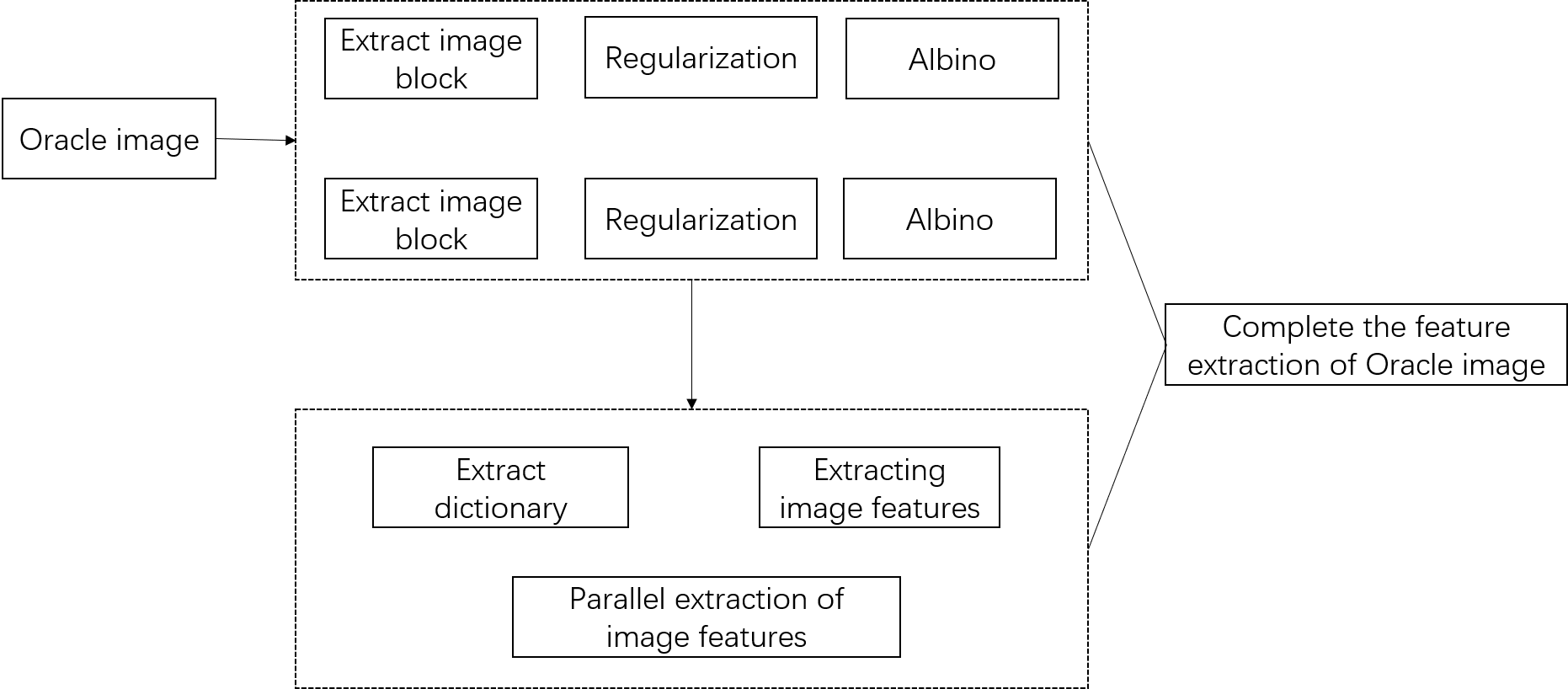### 2.2 Research on Image Feature Preprocessing of Oracle Bone Inscriptions

On the basis of the oracle bone inscription image feature extraction, the extracted oracle bone inscriptions’ image features show that the general image is complex, and there are some differences in the signal and the coordinate points in the image. Therefore, before the automatic classification of oracle images, it is necessary to preprocess the extracted oracle image features to provide consistent classification data for subsequent classification and reduce the difficulty of image classification. First, pretreatment is carried out using the CS method. CS is a new theoretical model that can realize compression while sampling. The preprocessing sampling process of CS is shown in Fig. 3. The theoretical framework of CS mainly includes three important contents: (1) sparse representation of signals, (2) design of an observation matrix, and (3) signal reconstruction. The pretreatment process is shown in Fig. 3.

##### Fig. 3. C S image preprocessing and sampling process.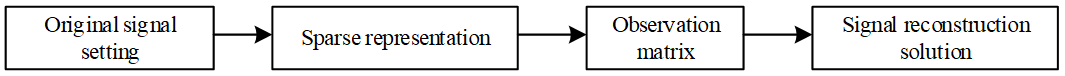In this method, if the signal $\mathrm{Y}\in \mathrm{H}^{\mathrm{n}}$ is sparse or compressible on an orthogonal basis or compact frame$\varphi$, the transformation coefficient is obtained by:

##### (6)
$\mathrm{G}=\varphi ^{\mathrm{T}}\mathrm{Y}$

where $\mathrm{G}$ represents an equivalent or approximated sparse representation of the feature points in the oracle image. Then, according to the determined feature representation results, we design a stable$\mathrm{M}\times \mathrm{N}$ dimensional observation matrix ℉, which is not associated with the exchange base. Then, we observe $\mathrm{G}$ and obtain Eq. (7):

##### (7)
$\mathrm{D}=\mathrm{℉}\varphi ^{\mathrm{T}}\mathrm{Y}$

The oracle image feature observation matrix process is $\rho$represented as a signal non-adaptive observation through the matrix$\sigma ^{CS}$:

##### (8)
$\rho =\sigma ^{CS}Y,\,\,\,\sigma ^{CS}=\mathrm{℉}\varphi ^{\mathrm{T}}$

where $\sigma$ is the operator representing the CS algorithm.

Finally, solving for the exact or approximate value of $\overset{˜}{X}$ with the optimization problem in the sense of 0-norm yields:

##### (9)
$\overset{˜}{X}=argmin\left\| \varphi ^{\mathrm{T}}\mathrm{X}\right\| ,\,\,S.T\sigma ^{CS}=\varphi ^{T}$

According to this calculation, the feature points of the oracle image are observed to confirm the effectiveness of the feature points. Then, the oracle image feature signal is compressed; that is, the oracle feature CS signal is sparse under a certain base. A sparse signal is defined as the exchange coefficient vector which satisfies the following formula:

##### (10)
$\left\| G\right\| =\left(\sum \left| \vartheta _{i}\right| ^{Q}\right)\leq R$

where $\vartheta _{i}$ represents the sparse signal point of the oracle image Q, and $R$represents the threshold of the image feature point.

Based on this, the preprocessing of oracle image features was realized. All oracle image feature points are regarded as a sparse signal, and then sparse processing is carried out to realize the overall preprocessing. The results are as follows:

##### (11)
$\mathrm{H}=\mathrm{L}\sum _{\mathrm{i}=1}^{\mathrm{n}}\mu _{\mathrm{i}}\varphi ^{\mathrm{T}}$

$\mu _{\mathrm{i}}$ represents the image feature point basis vector, H represents the preprocessing results, $\varphi$ is an orthogonal basis or compact frame, and L represents the consistency coefficient of the sparse signal.

## 3. Research on Automatic Classification of Oracle Images based on Deep Learning

### 3.1 Target Alignment of Feature Points in Oracle Image

Target alignment is a technical measure to locate a target object . A simple method is to take the mean value of the coordinates corresponding to each local feature point as the center point of the target. However, there are still many problems in the process of actual oracle image feature processing. Therefore, it is necessary to align the feature points of oracle images before classification to reduce the classification error of subsequent oracle images.

<New paragraph>If all feature points are averaged directly, the problem of center point offset will occur. At the same time, the local areas corresponding to these feature points have much overlap and have no value listed separately. Therefore, we integrated the feature points into one feature point, and its coordinate point is the coordinate mean value of these feature points. The centers of six feature points were used to describe the center of the target object of the feature points of the whole oracle image, in which the position of the feature points will change greatly. Thus, the calculation results of the center point are greatly affected, and the following results are obtained:

##### (12)
$\delta _{x}=\frac{1}{N}\sum _{i=1}^{N}W\left(i\right)x,\delta _{y}=\frac{1}{N}\sum _{i=1}^{N}W\left(i\right)y$

where $\delta _{x}$ and $\delta _{y}$ represent the coordinates of the center point of the oracle image feature target, N represents the number of feature points present in the graph, and $W\left(i\right)x$ and $W\left(i\right)y$ represent the coordinates of these present feature points.

In the alignment process, the key parts of the oracle image features are included, and the expression forms of the key features are as follows:

##### (13)
$\left\| Q\left(i\right)x\right\| _{0}\leq 1，\,\,\,\left\| Q\left(i\right)y\right\| _{0}$

$Q\left(i\right)x$ and $Q\left(i\right)y$ represent the image point coordinates of the characteristic points extracted from the oracle bone text image, and the points that do not appear in the coordinate are removed:

##### (14)
$\omega _{i}=\mathit{\arctan }\left(-\left(\tau _{x}-k_{y}\right),\tau _{x}-k_{y}\right)$

Based on the elimination of non-key oracle image feature points, the target alignment of oracle feature points is realized, and the alignment equation is:

##### (15)
$\left| \beta -\gamma _{Q}\left(i\right)\right| <M_{0}$

In Eq. (15), $M_{0}$ represents the key threshold variable with feature points aligned, and $\beta$ represents the number of threshold feature points.

### 3.2 Design of Oracle Image Automatic Classifier based on Deep Learning

After the key points of anoracle image are aligned, we useda deep learning algorithm to design an automatic classifier for anoracle image to realize the final classification. In the field of deep learning, model migration refers to the migration of the trained model to the target task. Because a large model usually needs to be trained in deep learning, it needs much label data to prevent over fitting . However, sometimes, the target data does not have much label data, but the target task has similar characteristics to other tasks with a large amount of data, so model migration can be carried out .

<New paragraph>For example, the source task is image recognition. Image recognition has a large amount of labeled data, such as ImageNet data, which can be used to train a model. Target tasks can be similar, such as specific target recognition or detection, or target recognition in special scenes. Therefore, in the design of the automatic classifier, we first learned the preprocessed oracle images using transfer learning. The goal of transfer learning is to solve the classification problem when the training data from the source domain is different from the data in the target domain.

<New paragraph>Transfer learning methods mainly have two categories. The first kind is better for the classification problem of the target domain by learning to add different weights to the source domain data. The second type finds a common feature space, maps the source domain and target domain data into this feature space, learns through the features of the source domain mapping, and then is tested with the features of the target domain mapping to complete the classification of the target domain data. For the second kind of method, we mainly use linear mapping or a kernel method to minimize the distribution of source domain data and target domain data under the assumption that the target task does not have labeled data. In this way, the model needs unsupervised adding of the information of the target task data during training so that the model can adapt to the target task.

The data in the oracle image feature are set as the training data in the source domain, including training sample data $s_{i}$. We used $s_{ix}$ to represent oracle image sample i, and the sample data are dimensional vector data D. We used $s_{iy}$ to represent the oracle image data after the label and $C_{s}$ to represent the number of villas.<note: ambiguous>We set the target domain as:

##### (16)
$\alpha _{i}=\left\{\epsilon _{i}\left| i=1,2\ldots N\right.\right\}$

where $N$ represents the number of samples trained on the oracle images.

Then, given target domain data $X_{t}$and source domain data $X_{i}$, usually, their probability density distribution is different when they are taken from different datasets. In order to reduce the difference of distribution, we hope that in the migrated feature space, the probability distribution of source domain features and target domain features are as close as possible. In order to achieve this goal, the MMD criterion was used to measure the difference of distribution. This criterion acts on the previous layer of the classification layer (that is, the final feature output of the network) and is used to measure the difference of the output features of the source domain data and the target domain data after the feature transformation of the network. The equation of MMD is:

##### (17)
$\mathrm{MMD}\left(X_{t},X_{i}\right)=\left\| s_{ix}-s_{iy}\right\| ^{2}$

where $\left\| s_{ix}-s_{iy}\right\| ^{2}$ is the square of mmd, and the inner product can be obtained by simplifying the equation.

Since the data center (first-order statistics) represents the average characteristics of the image and is a way to represent the data distribution, the MMD criterion is adopted so that the centers of the source domain data and the target domain data can be closer after network transformation. Aconvolution neural network was used to design the automatic classifier of an oracle image. A convolutional neural network is a hierarchical structure model. A standard convolutional neural network is generally composed of a data input layer, convolution layer, pooling layer, full connection layer, and output layer. The input is the original data, such as an image, original audio data, etc. The data input layer is used to preprocess the original input data, such as deaveraging, normalization, etc. Using data preprocessing can avoid affecting the training effect due to too many data deviations.

<New paragraph>The convolutional neural network extracts the high-level semantic information layer by layer from the original data input layer and abstracts it layer by layer (that is, feedforward operation). The convolutional neural network formalizes the training task objective into the objective function model at the output layer. Through the back-propagation algorithm, the error value between the predicted value and the real value is transmitted back from the last layer step by step to update the parameters between levels and fed back again after updating the parameters. In this way, the process is repeated until the function model convergesto realize the classification training of the network model.

If matrix x is used to represent the input image, the convolution kernel is matrix w, and bias is b, then the calculation of a convolution surface can be expressed as:

##### (18)
$\mathrm{U}=\mathrm{x}\times \mathrm{w}+\mathrm{b}$

If the input is multiple channels (that is, if there are D images $\left(\mathrm{x}_{1},\mathrm{x}_{2},\ldots \mathrm{x}_{\mathrm{D}}\right)$), the corresponding convolution kernel is $\left(\mathrm{w}_{1},\mathrm{w}_{2},\ldots \mathrm{w}_{\mathrm{D}}\right)$, the bias is b, and the convolution surface is:

##### (19)
$\mathrm{U}=\sum _{\mathrm{i}=1}^{\mathrm{D}}\mathrm{x}_{\mathrm{i}}\times \mathrm{w}_{\mathrm{i}}+\mathrm{b}$

During a convolution operation, the scale of the output characteristic image becomes smaller and distorted compared with the input image. The more layers, the smaller the image is, resulting in the inability to continuously increase the number of convolution layers.

The edge of the input feature matrix will be calculated less than the elements inside the matrix<note: ambiguous>, so the edge data will be continuously lost. The data at the first edge will be discarded after being used only once so that the influence of the edge pixels on the network is less than that of the pixels in the center, which is not conducive to feature extraction. Therefore, the padding operation generally adds several pixels to the peripheral edge of the input characteristic matrix so that the sliding unit is a certain step from the initial position to the end position. We do this to maintain the size of the characteristic image after the convolution operation.

<New paragraph>The padding operation not only maintains the consistency of the input dimension and output dimension of the convolution layer, but also maintains the boundary information of the feature map, which improves the reference degree of the boundary information to a certain extent. Therefore, based on this analysis, an activation function is introduced to improve the boundary information of the oracle image, and the equation is as follows:

##### (20)
$\text{sigm}\left(\mathrm{x}\right)\frac{1}{1+\mathrm{r}^{-\mathrm{x}}}$

The range of the sigmoid function is (0,1), which can map the output to the interval (0,1). It is optimized and stable and can be used as the output layer.

Because the saturation gradient value of the sigmoid function is very small, the gradient of the algorithm in back-propagation is transmitted to the front layer in a multiplicative way<note: ambiguous>. Therefore, when the number of network layers is deep, the value of gradient back-propagation to the front layer is very small, and the weight parameters cannot be effectively updated in time, which makes iteration difficult and makes it easy to cause gradient disappearance. The model also has difficulty converging, resulting in training problems. Therefore, the linear rectification function is introduced to correct this problem, and its calculation formula is:

##### (21)
$\text{ReLU}\left(\mathrm{x}\right)=\max \left(0,\mathrm{x}\right)$

Compared with sigmoid and tanh functions, the ReLU functioncan converge more quickly in random gradient descent, and the expression of the ReLU function can be realized more simply. The ReLU function provides the sparse expression ability to a neural network and increases the sparsity of the network. To a certain extent, the greater the sparsity, the more representative the features extracted by the network are, and the faster the calculation speed is. However, with continuous training, a small part of the input features will fall into the hard saturation area. As a result, the corresponding weight parameters cannot be updated, which means the phenomenon of neuron death.'' LeakyReLU with a leakage linear rectifier improves the death characteristics of ReLU, but at the same time, it loses some sparsity, as shown in the formula:

##### (22)
$\operatorname{LeakyReLU}(\mathrm{x})=\left\{\begin{array}{c} x, x>0 \\ 0 \text { ó }<0 \end{array}\right.$

where x represents a constant, and $\nexists$ represents the leakage correction coefficient. Finally, combining the characteristics, the maximum output is:

##### (23)
$\operatorname{ELU}(\mathrm{x})=\left\{\begin{array}{c} x, x>0 \\ a\left(\mathrm{r}^{\mathrm{x}}-1\right) \end{array}\right.$

The maximum output function, MAXOUT, gives the following:

##### (24)
$\text{Maxout}\left(\mathrm{x}\right)=\max \left(\mathrm{x}_{1},\mathrm{x}_{2},\ldots \mathrm{x}_{\mathrm{n}}\right)$

Finally, the designed oracle text image automatic classifier model is:

##### (25)
$\text{softmax}\left(\mathrm{x}_{1},\mathrm{x}_{2},\ldots \mathrm{x}_{\mathrm{n}}\right)=\frac{1}{\sum _{\mathrm{i}=1}^{\mathrm{n}}\exp \left(\mathrm{x}_{\mathrm{i}}\right)_{\mathrm{m}\times \mathrm{n}}}$

The oracle image feature data is input into the constructed deep learning automatic classifier, the output is the final result of classification, and the final oracle image automatic classification is completed.

## 4. Experimental Analyses

### 4.1 Design of Experimental Scheme

In order to verify the feasibility of the automatic classification algorithm of oracle images designed in this study, experimental analysis was carried out. In the experiment, 500 oracle images from the MySQL database were selected as the sample images. The algorithm program was designed via the Microsoft Professional programming system to lay the foundation for subsequent experiments. The specific experimental data are shown in Table 1.

In the experiment, the algorithm in this paper was compared with classification methods in the literature [9,10]. The feature extraction error and image classification accuracy of the sample oracle image were adopted as the experimental indicators to verify the effect and effectiveness of different methods in oracle image classification. The oracle image used in the feature extraction is shown in Fig. 4.

##### Table 1. Experimental Parameters.
 Parameter Content Number of oracle images/piece 500 oracle text image size 256*256 oracle text image resolution/dpi 1024 pattern noise /dB [-4-2] Classification group/group 2 class interval /s 1.5 Number of iterations/times 100
##### Table 2. Performance comparison between proposed method and existing method.
 Item Proposed method (%) Cross modal depth measurement learning method (%) Capsule network method (%) Data set 1 96.4 87.2 86.9 Data set 2 93.1 85.4 85.7 Data set 3 90.5 82.2 80.7 Data set 4 90.1 79.6 76.9 Data set 5 86.8 77.4 75.4 Average accuracy 91.38 82.36 81.12

### 4.2 Experimental Result

We extracted the features in the sample oracle image in Fig. 4. The methods in this paper and the literature [7,8] were used to extract the features of the sample oracle image. The error of the feature extraction of the sample oracle image by the three methods was analyzed. The results are shown in Fig. 5.

By analyzing the experimental results in Fig. 5, it can be seen that there are certain differences in the error of feature extraction of the sample oracle image by using the three different methods. As can be seen from Fig. 5(a), the feature points of the sample oracle image can be completely extracted by the proposed algorithm, while some feature points in the other two methods cannot be completely extracted. It can be seen from Fig. 5(b) that although the errors of the three methods are within a reasonable range, in the overall curve trend, the feature extraction error of the proposed algorithm is the lowest, which verifies the feasibility of the proposed method.

We then analyzed the classification accuracy of the three methods for 500 sample oracle images, and the results are shown in Fig. 6. By analyzing the experimental results in Fig. 6, it can be seen that there are certain differences in the accuracy of the 500 oracle images classified by the three methods. The classification accuracy of our algorithm for the 500 oracle images was always higher than 90%, while the classification accuracy of the other two methods was always lower than our method’s and fluctuated greatly. Therefore, it can be seen that the proposed algorithm is feasible.

In order to further prove the feasibility and superior performance of the proposed method, the proposed algorithm was compared with the cross modal depth measurement learning method and capsule network method in real datasets. According to the results, the proposed method is more accurate than the other algorithms in each dataset. The proposed method has about 10% higher average accuracy than the other two algorithms.

##### Fig. 5. Error extraction of different methods.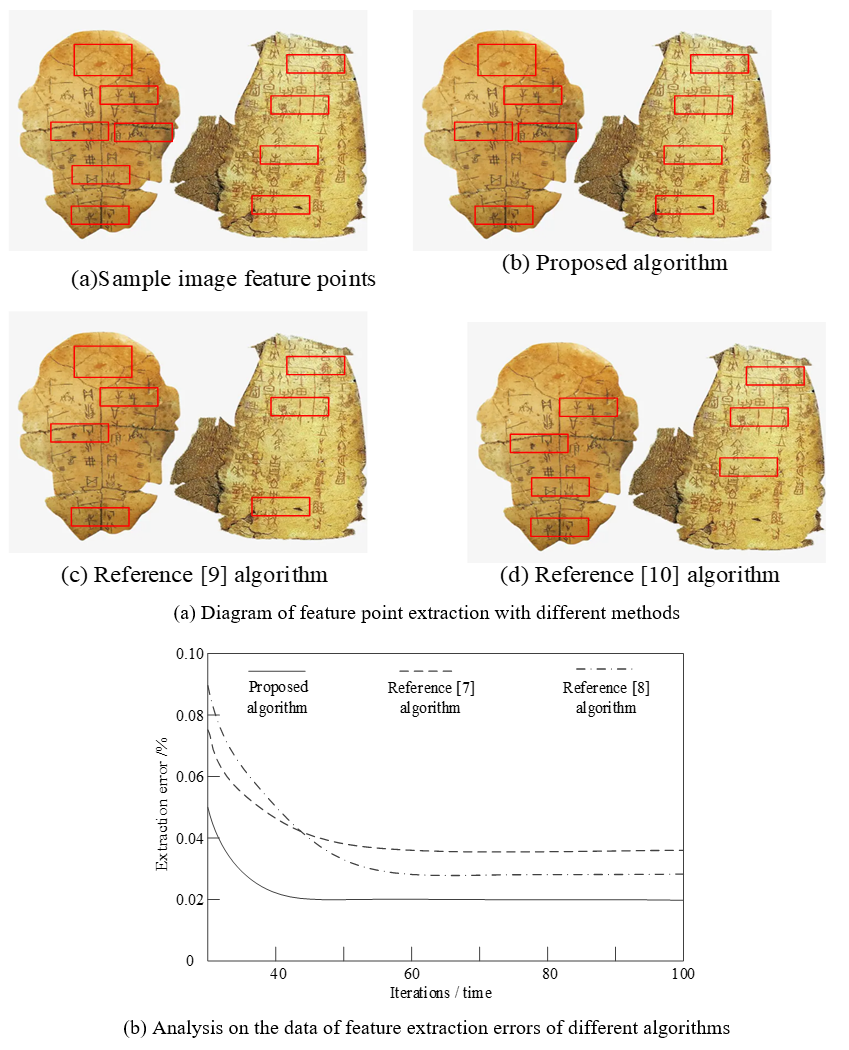##### Fig. 6. Analysis of oracle image classification accuracy in different methods.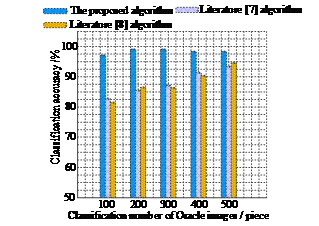## 5. Conclusion

In order to improve the efficiency of oracle image classification, we designed an automatic oracle image classification method based on deep learning. The clustering center of an oracle image was determined using the k-means algorithm, and the oracle image features were output through the feature mapping function to realize the feature extraction of an oracle image. The dimension of the oracle image was reduced by the CS algorithm, and the CS signal of an oracle feature was sparse under a certain base.

<New paragraph>The centers of six feature points in the feature were used to describe the center of the feature points of the whole oracle image, to determine the position change state of the feature points, and to characterize the key features of the image to realize the target alignment of the feature points of oracle image. With a transfer learning model, the image feature data was learned, and the automatic classifier of oracle images was constructed through a convolution neural network to realize the automatic classification of oracle images. The results show that the proposed method can effectively reduce the error of image feature extraction and improve the classification accuracy. At the level of theoretical contribution, the results of this research also provide an exploration direction for the optimization route of oracle image classification technology.

### ACKNOWLEDGMENTS

The research was supported by the Phased Achievements of Humanities and Social Sciences Funded by Youth Project of Ministry of Education,” A Study on Colleting Sorting and Visually Constructing Handwritten Characters of Oracle Bone Inscriptions” (Project No: 21YJCZH118); Phased Achievement of Henan Philosophy and Social Science Planning Project,” Research on Modular Design of Oracle” (Project No: 2020BYS027); and Phased Achievement of Henan Philosophy and Social Science Planning Project,” A Study on Colleting and Sorting Handwritten Characters of Oracle Bone Inscriptions and its Visual Dynamics Construction” (No: 2021BYS031).

### REFERENCES

1
Xiong. J, Liu. G, Liu. Y, Liu. M. Oracle Bone Inscriptions information processing based on multi-modal knowledge graph,'' Computers & Electrical Engineering, vol. 92, no. 6, pp. 107173, 2021.2
Wang. J.X. The Pattern of Religious Culture: Toward a New Theory for China's Ethnological and Anthropological Studies,'' Nationalities Research in Qinghai, vol. 4, pp. 1-14, 2007.3
Procopio. N, Hopkins. R, Harvey. VL, Buckley, M. Proteome variation with Collagen yield in ancient bone,'' Journal of Proteome Research, vol. 20 no.3, pp. 1754-1759, 2021.4
Bejiga. MB, Melgani. F, Vascotto. A. Retro-remote sensing: Generating images from ancient texts,'' IEEE Journal of Selected Topics in Applied Earth Observations and Remote Sensing, vol. 12, no. 3, pp. 950-960, 2019.5
Wang. K, Yi. Y, Tang. Z, Peng. J. Multi-scene ancient Chinese text recognition with deep coupled alignments,'' Applied Soft Computing, vol. 108, no. 4, pp. 107475, 2021.6
Chen. CM, Chang. C. A Chinese ancient book digital humanities research platform to support digital humanities research,'' The Electronic Library, vol. 37, no. 2, pp. 314-336, 2019.7
Zhang. YK, Zhang. H, Yong-Ge. L, Cheng. L, Oracle character recognition based on cross-modal deep metric learning,'' ACTA AtuomaticSinica, vol. 47, no. 4, pp. 791-800, 2020.8
Lu. X, Cai. H, Lin. L, Recognition of Oracle radical based on the capsule network,'' CAAI transactions on intelligent systems, vol. 15, no. 2, pp. 243-254, 2020.9
Chen. L, Lyu. B, Tomiyama. H, Meng. L. A method of Japanese ancient text recognition by deep learning - Sciencedirect,'' Procedia Computer Science, vol. 174, pp. 276-279, 2020.10
Liu Z. “Research on the Application of Green Landscape Buildings in the Sustainable Development of Ancient Villages - Take Ancient Villages in Southwest China for Example,” Landscape Architecture, vol. 787, no. 1, pp. 012066, 2021.11
Ashokkumar. P, Shankar. SG, Srivastava. G, Maddikunta. PK, Gadekallu. TR. A two-stage text feature selection algorithmfor improving text classification,'' Transactions on Asian and Low-Resource Language Information Processing, vol. 20, no. 3, pp. 1-19, 2021.12
Gupta. U, Gupta. D. Kernel-target alignment based fuzzy lagrangian twin bounded support vector machine,'' International Journal of Uncertainty, Fuzziness and Knowledge-Based Systems, vol. 29, no. 5, pp. 677-707, 2021.13
Maliar. L, Maliar. S. Deep learning classification: Modeling discrete labor choice,'' Journal of Economic Dynamics and Control, vol. 135, pp. 104295, 2022.14
Algan. G, Ulusoy. I, Image classification with deep learning in the presence of noisy labels: A survey. Knowledge-Based Systems'', vol. 215, no. 3, pp. 106771, 2021.## Author

Yingjie Qiao, associate professor, School of Art and Design, Luoyang University of Science and Technology, member of Henan Artists Association. Field of Research: art design, oracle bone inscriptions. She has published more than 10 articles in academic journals.

Lizhi Xing, associate professor, School of Art and Design, Luoyang University of Science and Technology, member of Chinese Artists Association. Field of Research: art design, oracle bone inscriptions. He has published more than 20 articles in academic journals.